Courses

Basic Trigonometry Concepts Notes | Study Quantitative Aptitude (Quant) - GMAT

GMAT: Basic Trigonometry Concepts Notes | Study Quantitative Aptitude (Quant) - GMAT

The document Basic Trigonometry Concepts Notes | Study Quantitative Aptitude (Quant) - GMAT is a part of the GMAT Course Quantitative Aptitude (Quant).
All you need of GMAT at this link: GMAT

What is Trigonometry?

Trigonometry is the branch of mathematics that deals with the study of relationships between sides and angles of a triangle. It is derived from the Greek word, Tri meaning three and Gon means Angle and Metron means Measure.

• The ratio of the lengths of two sides of a right-angled triangle is called a trigonometric ratio.
• The trigonometric ratios of a triangle are also called the trigonometric functions. There are six trigonometric ratios.
• Sine, cosine, and tangent are 3 important trigonometric functions and are abbreviated as sin, cos, and tan.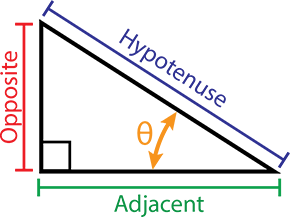• Consider a right-angled triangle ABC, where the longest side is called the hypotenuse, and the sides opposite to the hypotenuse are referred to as the adjacent and opposite sides.
AC = Hypotenuse of triangle
AB = Side adjacent to angle A
BC = Side opposite to angle A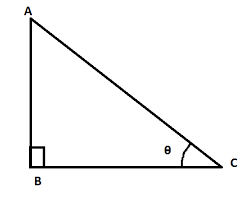Trigonometric Ratios of Right-Angled Triangle ABC with ∠B = 90°
(i) sin A = Sine of ∠A = (Side opposite to ∠A / hypotenuse) = (BC/AC)
(ii) cos A = Cosine of ∠A = (Side adjacent to ∠A / hypotenuse) = (AB/AC)
(iii) tan A = Tangent of ∠A = (Side opposite to ∠A / Side adjacent to ∠A) = (BC/AB)
(iv) cosec A = Cosecant of ∠A = Hypotenuse / Side opposite to ∠A = 1/sin A
(v) sec A = Secant of ∠A = Hypotenuse / Adjacent side = 1/cos A
(vi) cot A = Cotangent of ∠A = Adjacent side / Opposite side = 1/tan A

• The trigonometry angles which are commonly used in trigonometry problems are  0°, 30°, 45°, 60° and 90°.
• The trigonometric ratios such as sine, cosine and tangent of these angles are easy to memorize.
Example: In a right-angled triangle:
⇒ Sin θ = Perpendicular/Hypotenuse
or θ = sin-1 (P/H)
⇒ Similarly,
θ = cos-1 (Base/Hypotenuse)
θ = tan-1 (Perpendicular/Base)

Trigonometric Ratios of Specific Angles

• The trigonometric ratios of some special angles, i.e. 0°, 30°,45°,60°,90° follow a pattern and are easy to remember.
• Identifying and remembering these patterns helps in solving problems involving these angles.
Table: Trigonometric Ratios of Specific Angles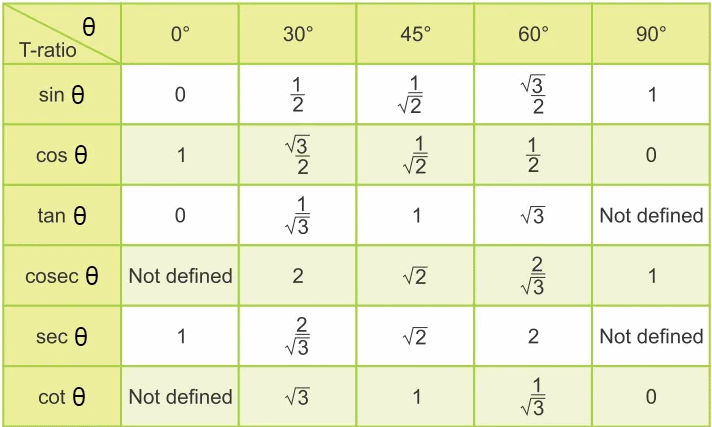• Two angles are said to be complementary if their sum is 90°. Thus ϴ and (90 – ϴ) are complementary angles.
• Representing complementary angles in terms of these standard angles helps in solving a complex problem involving trigonometric ratios.

Try yourself:Value of tan 30°/cot 60° is:

Trigonometric Ratios of Complementary Angles

• Sin (90° – A) = Cos A
• Cos (90° – A) = Sin A
• Tan (90° – A) = Cot A
• Cot (90° – A) = Tan A
• Sec (90° – A) = Cosec A
• Cosec (90° – A) = Sec A

Basic Trigonometric Identities

• sin2θ + cos2θ = 1
• tan2θ + 1 = sec2θ
• cot2θ + 1 = cosec2θ
• Sinθ /Cosθ = tanθ
• Cosθ / Sinθ = cotθ
• sin 2θ = 2 sin θ cos θ

• cos 2θ = cos²θ – sin²θ

• tan 2θ = 2 tan θ / (1 – tan²θ)

• cot 2θ = (cot²θ – 1) / 2 cot θ

Try yourself:The value of sin θ and cos (90° – θ)

Sum and Difference Identities

For angles u and v, we have the following relationships:

• sin(u + v) = sin(u)cos(v) + cos(u)sin(v)
• cos(u + v) = cos(u)cos(v) – sin(u)sin(v)
• tan(u+v) = (tan(u) + tan(v)) / (1−tan(u) tan(v))
• sin(u – v) = sin(u)cos(v) – cos(u)sin(v)
• cos(u – v) = cos(u)cos(v) + sin(u)sin(v)
• tan(u-v) = (tan(u) − tan(v)) / (1+tan(u) tan(v))

Value change with change of angle by π/2 or π or negative sign

When the angle of θ is changed by π/2 or by π the values of the trigonometric functions change in simple ways.

1. Change by π/2: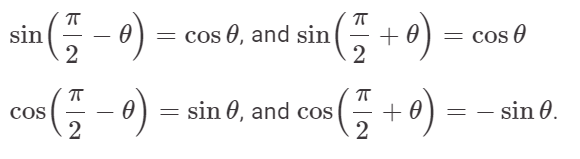2. Change by π: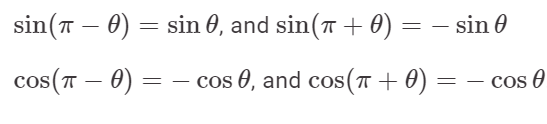3. Change by Sign:

sin(−θ) = −sinθ, and cos(−θ)=cosθ.

Two Special Triangles

• There are two special triangles we need to know 45°-45°-90° and 30°-60°-90° triangles. They are depicted in the figures below.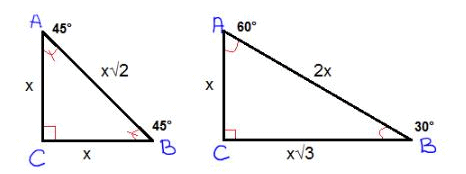• The figures show how to find the side lengths of those types of these special triangles.
• In a 45-45-90 triangle ABC shown above, ratio of side AC:BC:AB = 1:1:1/√2
• In a 60-30-90 triangle ABC shown above, ratio of sides AC:BC:AB = 1: 1/√3: 2

Application of Trigonometry - Heights and Distances

In this problem area, an object stands upright perpendicularly on the surface and from a distance the object is observed. If the angle of elevation is known along with one of the height or distance to the object, the other can be known.

1. Angle of Elevation

When the observer looks up to the top of a tall object, the angle made by the horizontal line through point of observation and the line from the top of the object to the point of observation is the angle of elevation.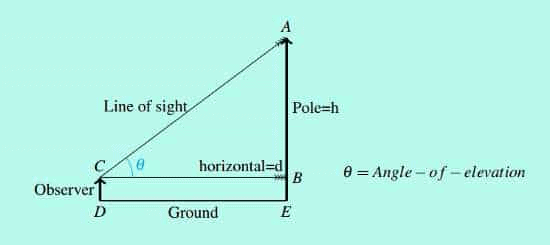We have here, h/d= tanθ

2. Angle of Depression

When an observer looks down from the top of a tall object, we get angle of depression.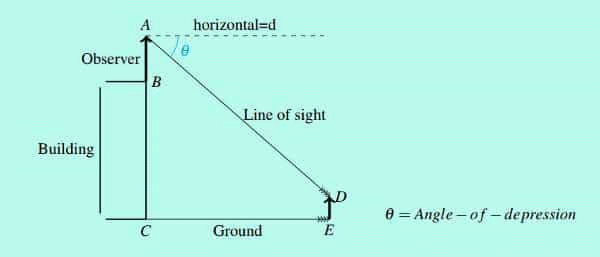Example: From a point A on the bridge over a river, the angles of depression of the banks on the opposite sides of the river are 30º and 45º respectively. If the bridge is at a height of 9m above the river surface, find how wide is the river.

Width of the river is DC. In triangle △ABC, tan 30º= AB/BC300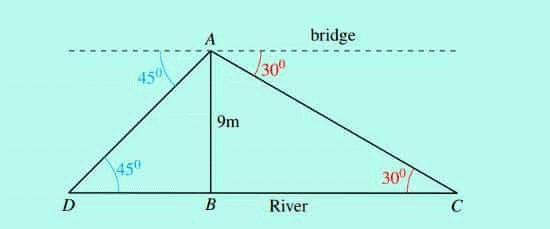Solution: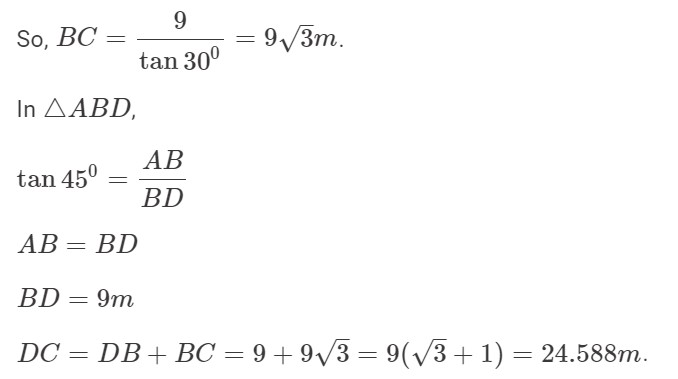Important Solved Examples

Let us look at some examples to understand the concept better:

Example 1: Anil looked up at the top of a lighthouse from his boat and found the angle of elevation to be 30º. After sailing in a straight line 50 m towards the lighthouse, he found that the angle of elevation changed to 45º. Find the height of the lighthouse.
a) 25
b) 25√3
c) 25(√3-1)
d) 25(√3+1)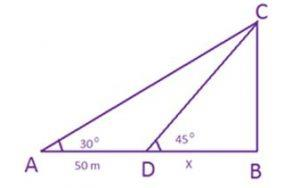If we look at the above image, A is the previous position of the boat. The angle of elevation from this point to the top of the lighthouse is 30 degrees. After sailing for 50 m, Anil reaches point D from where the angle of elevation is 45 degrees. C is the top of the lighthouse.

Let BD = x
⇨ Now, we know tan 30 degrees = 1/√3 = BC/A
⇨ Tan 45 degrees = 1
⇨  BC = BD = x
Thus, 1/√3 = BC/AB = BC/(AD+DB) = x/(50 + x)
Thus x (3 -1) = 50 or x= 25(3 +1) m.

Try yourself:From a point P on a level ground, the angle of elevation of the top tower is 30°. If the tower is 100 m high, the distance of point P from the foot of the tower is:

Example 2: An aeroplane when flying at the height of 3125 m from the ground passes vertically below another plane at an instant when the angles of elevation of the two planes from the same point on the ground are 30º and 60º, respectively. Find the distance between the two planes at that instant.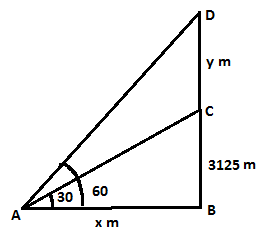Let C and D be the two aeroplanes, and A be the point of observation. Then,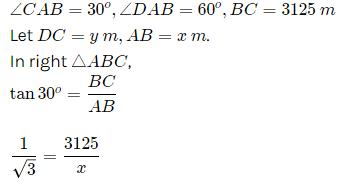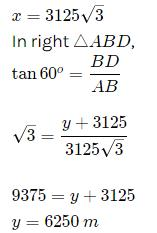Therefore, the distance between the two planes is 6250 m.

Try yourself:The angle of elevation of a ladder leaning against a wall is 60° and the foot of the ladder is 4.6 m away from the wall. The length of the ladder is:

Example 3: An airplane flying at 3000 m above the ground passes vertically above another plane at an instant when the angle of elevation of the two planes from the same point on the ground are 60º and 45º respectively. The height of the lower plane from the ground is:
a) 1000√3 m
b) 1000/√3 m
c) 500 m
d) 1500(√3+1)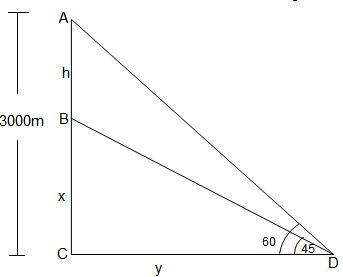Let the higher plane fly such that at point A the angle of elevation from point D is 60º.
Let the height of the plane flying at a lower level be CD = y (since tan 45º= 1)
tan 60º = 3000/y
⇨ √3 = 3000/y
⇨ y =  3000/√3 = 1000√3

Example 4: What is the value of (1-CosA) / (1+CosA) given that tan A = ¾?

a) 1/4

b) 9/16

c) 1/9

d) 1/3

Solution:

We know that tan A = opposite side / adjacent side = 3/4

Therefore, opposite side = 3, adjacent side = 4 and the hypotenuse = 5

Hence, cos A = 4/5 Therefore, (1-Cos A) / (1 + Cos A) = (1- (4/5)) / (1 + (4/5)) = 1/9

Example 5: From a point on a bridge across a river, the angles of depression of the banks on opposite sides of the river are 30 degree and 45 degree respectively. If the bridge is at a height of 3 m from the banks, find the width of the river.

Solution: In the below figure, A and B represent points on the bank on the opposite sides of the river. P is a point on the bridge at a height of 3 m. Length of the side AB is the width of the river.

Now, AB = AD + DB

In right Δ APD, angle A = 30°

So, tan 30° = PD / AD

Or 1/ √3 = 3 / AD or AD = 3√3 m

Also, In right Δ PBD, angle B = 45°. So, BD = PD = 3 m

Now, AB = BD + AD = 3 + 3√3 m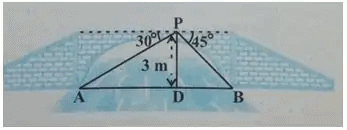Example 6: 3sinx + 4cosx + r is always greater than or equal to 0. What is the smallest value ‘r’ can take?

a) 5

b) -5

c) 4

d) 3

Ans: Choice A is the correct answer.

Sol: 3sinx + 4cosx ≥ -r
5(3/5sinx + 4/5cosx) ≥ -r
3/5 = cosA => sinA =4/5
5(sinx cosA + sinA cosx) ≥ -r
5(sin(x + A)) ≥ -r
5sin (x + A) ≥ -r
-1 ≤ sin (angle) ≤ 1
5sin (x + A) ≥ -5
rmin = 5

Example 7:  Sin2014x + Cos2014x = 1, x in the range of [-5π, 5π], how many values can x take?

a) 0

b) 10

c) 21

d) 11

Ans: Choice C is the correct answer.

Sol:

We know that Sin2x + Cos2x = 1 for all values of x.
If Sin x or Cos x is equal to –1 or 1, then Sin2014x + Cos2014x will be equal to 1.
Sin x is equal to –1 or 1 when x = –4.5π or –3.5π or –2.5π or –1.5π or –0.5π or 0.5π or 1.5π or 2.5π or 3.5π or 4.5π.
Cosx is equal to –1 or 1 when x = –5π or –4π or –3π or –2π or –π or 0 or π or 2π or 3π or 4π or 5π.
For all other values of x, Sin2014 x will be strictly lesser than Sin2x.
For all other values of x, Cos2014 x will be strictly lesser than Cos2x.
We know that Sin2x + Cos2x is equal to 1. Hence, Sin2014x + Cos2014x will never be equal to 1 for all other values of x. Thus there are 21 values.

Example 8: Consider a regular hexagon ABCDEF. There are towers placed at B and D. The angle of elevation from A to the tower at B is 30 degrees, and to the top of the tower at D is 45 degrees. What is the ratio of the heights of towers at B and D?

a) 1:√3

b) 1:2√3

c) 1:2

d) 3:4√3

Ans: Choice B is the correct answer.

Sol:

When you look at your reflection through a mirror, the image is at a distance equal to the distance between mirror and you. Now, think about what this has to with trigonometry.

Let the hexagon ABCDEF be of side ‘a’. Line AD = 2a. Let towers at B and D be B’B and D’D respectively.
From the given data we know that ∠B´AB = 30° and ∠D´AB = 45°. Keep in mind that the Towers B’B and D´D are not in the same plane as the hexagon.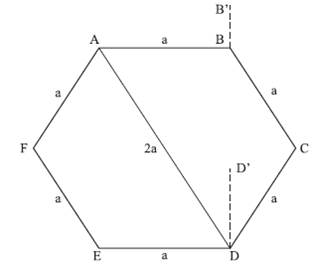In Triangle B’AB,
Tan∠B´AB = B´AB = B′B/AB = 1/3
=> B’B = a/√3
=> D’D = 2a
The ratio of heights = 1/√3 or 1/2√3

Choice B is the correct answer.

Conclusion

As we have seen, questions from trigonometry are based on mostly heights and distances and trigonometric identities. The questions above on height and distances are good representations of types of problems one can expect in the exams also. For solving Identities related questions, if we remember some of the main formulae, value of the angles for ratios, and Pythagorean triplets we should be good to go.

The document Basic Trigonometry Concepts Notes | Study Quantitative Aptitude (Quant) - GMAT is a part of the GMAT Course Quantitative Aptitude (Quant).
All you need of GMAT at this link: GMATUse Code STAYHOME200 and get INR 200 additional OFF Use Coupon Code

Top Courses for GMAT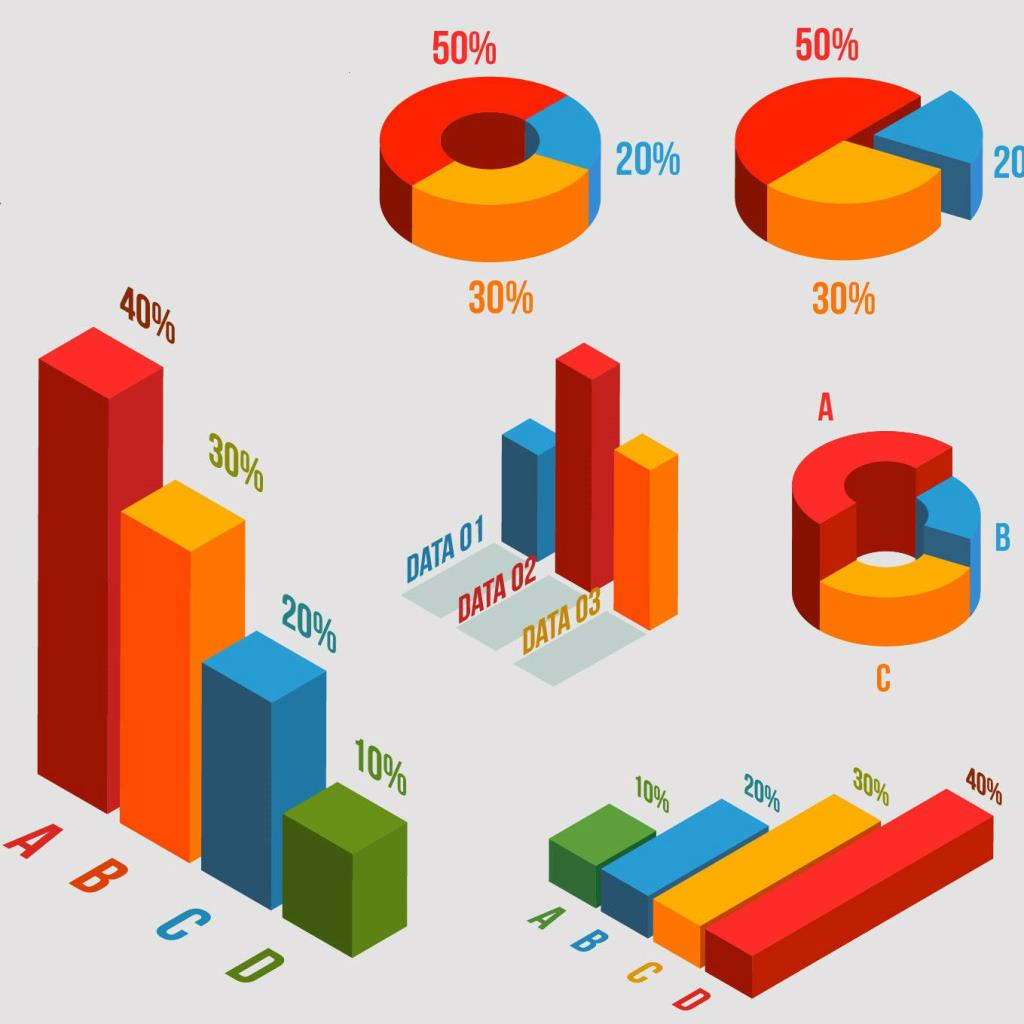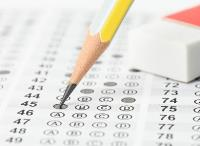Quantitative Aptitude (Quant)

134 videos|131 docs|131 tests

Top Courses for GMATTrack your progress, build streaks, highlight & save important lessons and more!

,

,

,

,

,

,

,

,

,

,

,

,

,

,

,

,

,

,

,

,

,

;